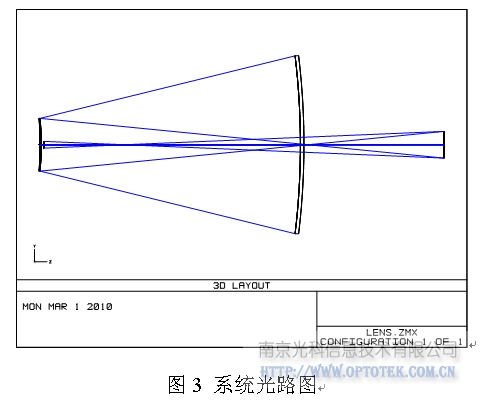目 录

如设计一个TMA系统，参数如下：

a1=0.33，a2=-0.5，b1=-2，b2=3，f=2000，孔径D=120的光学系统（光阑面为次镜）。

1）初始结构的计算

[code]

a1=0.33

a2=-0.5

b1=-2

b2=3

f=2000

curv(1)=b1*b2/(2*f)               #设置的是曲率，故需将公式做调整。

curv(2)=(b1+1)*b2/(2*f*a1)

curv(3)=(b2+1)/(2*f*a1*a2)

thic(1)=(1-a1)*f/(b1*b2)

thic(2)=a1*(1-a2)*f/(b2)

thic(3)=a1*a2*f

[/code]